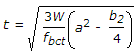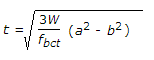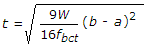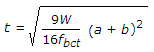# Civil Engineering - Structural Design Specifications

### Exercise :: Structural Design Specifications - Section 3

21.

Creep of concrete is assumed proportional to the stress if the stress does not exceed .......of the characteristic compressive strength

 A. 15% B. 20% C. 25% D. 33% E. 50%

Explanation:

No answer description available for this question. Let us discuss.

22.

The characteristic strength of concrete is the strength of material if its test result is not less than

 A. 2% B. 3% C. 4% D. 5% E. 10%

Explanation:

No answer description available for this question. Let us discuss.

23.

Pick up the correct statement from the following:

 A. Flange joints are preferably located at points of maximum stress B. The area of splice plates should be 5% in excess of flange elements spliced C. The C.G. of the flange splice should coincide with that of the element spliced D. For welding flange splice, complete penetration butt welds should be used E. None of these.

Explanation:

No answer description available for this question. Let us discuss.

24.

If a and b are the sides of a square column and its base, the thickness of the base may be calculated from the formula

 A.B.C.D.Explanation:

No answer description available for this question. Let us discuss.

25.

The minimum length of the side or diameter of the column base shall not be less than (where d is the minimum diameter of the column).

 A. (d + 75) mm B. 1.2 (d - 75) mm C. 1.5 (d + 75) mm D. 1.6 (d + 75) mm